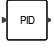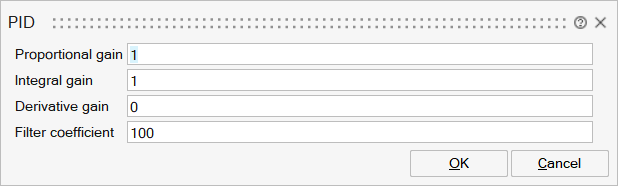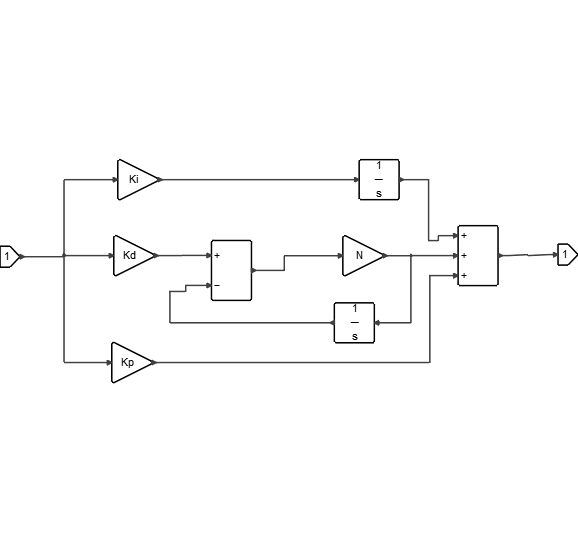# PID

This block is a PID (Proportional-Integral-Derivative) controller.Dynamical

## Description

The PID block implements a PID controller. The PID controller calculation (algorithm) involves three separate parameters: Proportional, Integral and Derivative. The Proportional value determines the reaction to the current error, the Integral value determines the reaction based on the sum of recent errors, and the Derivative value determines the reaction to the rate at which the error has been changing. The weighted sum of these three actions is used to adjust the process via a control element such as the position of a control valve or the power supply of a heating element.

## ParametersNameLabelDescriptionData TypeValid Values

Kp

Proportional gain

Real value. The value of the gain that multiplies the error.

Scalar

Ki

Integral gain

Real value. The value of the integral time of the error.

Scalar

Kd

Derivative gain

Real value. The value of the derivative time of the error.

Scalar

N

Filter coefficient

Real value. The value of the filter coefficient, which determines the pole location of the filter in the derivative action.

Scalar

## Ports

NameTypeDescriptionIO TypeNumber

Port 1

explicit

output

1

Port 2

explicit

input

1

NameValueDescription

always active

yes

direct-feedthrough

yes

PID block is direct-feedthrough unless the proportional gain (Kp) is zero (0).

zero-crossing

no

mode

no

continuous-time state

no

discrete-time state

no

## Diagram